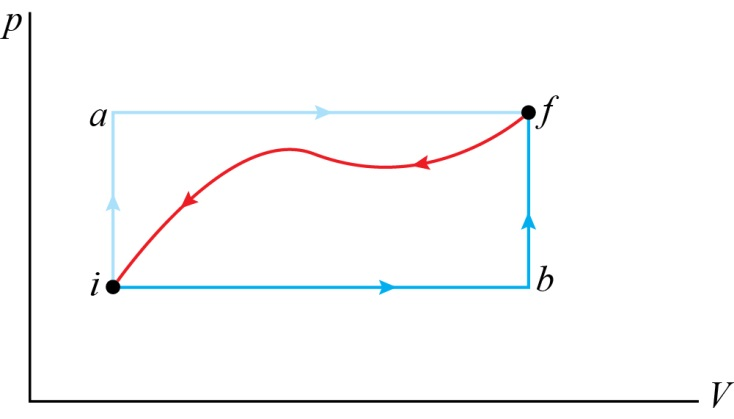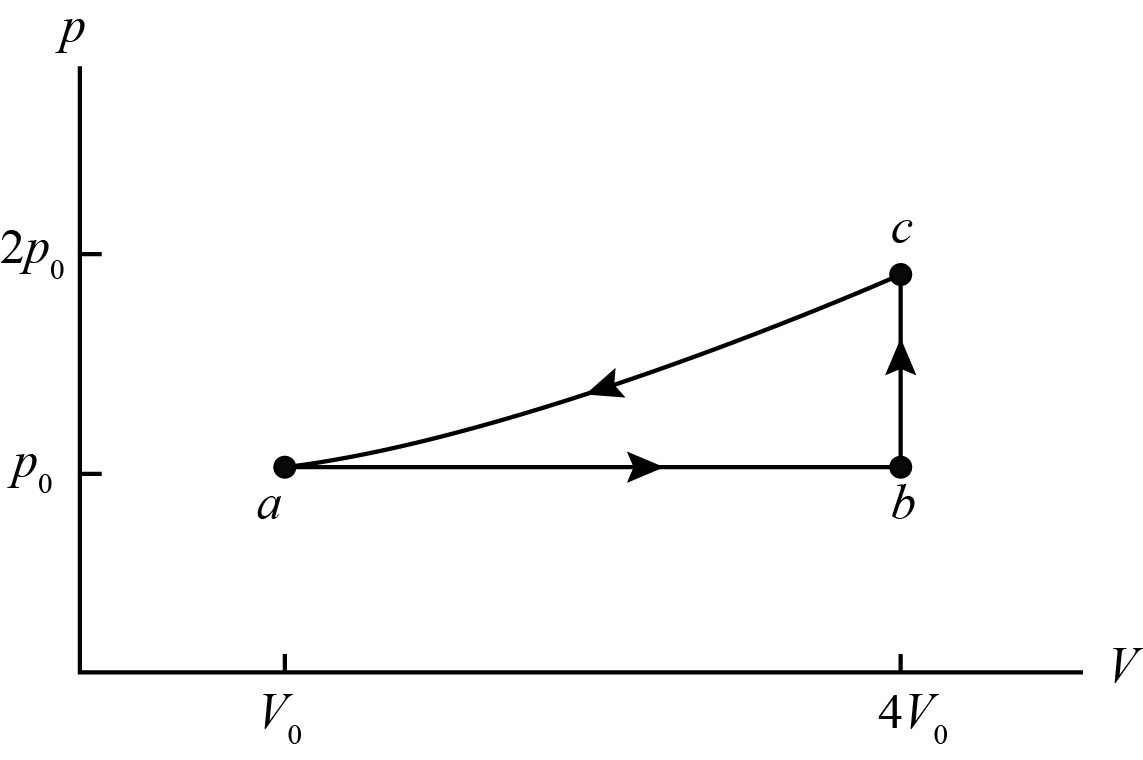×#### Thank you for registering.

One of our academic counsellors will contact you within 1 working day.

Click to Chat

1800-1023-196

+91-120-4616500

CART 0

• 0

MY CART (5)

Use Coupon: CART20 and get 20% off on all online Study Material

ITEM
DETAILS
MRP
DISCOUNT
FINAL PRICE
Total Price: Rs.

There are no items in this cart.
Continue Shopping• Complete JEE Main/Advanced Course and Test Series
• OFFERED PRICE: Rs. 15,900
• View Details

```Solved Examples on Thermodynamics

Question:1

When a system is taken from state i to state f along the path iaf in below figure, it is found that Q = 50 J and W = -20 J. Along the path ibf, Q = 36 J. (a) What is W along the path ibf? (b) If W = +13 J for the curved return path fi, what is Q for this path? (c) Take Eint,i = 10 J. What is Eint.f? (d) If Eint,b = 22 J, find Q for process ib and process bf.Concept:

For a thermodynamic system, in which internal energy is the only type of energy the system may have, the law of conservation of energy may be expressed as

Q + W = ΔEint

So, W= ΔEint -Q

And

Q= ΔEint – W

Here Q is the energy transferred (as heat) between the system and environment, W is the work done on (or by) the system and ΔEint is the change in the internal energy of the system.

By convention we have chosen Q to be positive when heat is transferred into the system and W to be positive when work is done on the system.

Solution:

A system is taken from i to state f along the path iaf, which as shown in below figure.(a) ΔEint along any path between these two points i and f  is,

ΔEint = Q+W

= (50 J)+(-20 J)

=30 J

To obtain the W along the path ibf, substitute 30 J for ΔEint, 36 J for Q in the equation W= ΔEint –Q, we get,

W= ΔEint –Q

= (30 J)-(36 J) = -6 J

Thus, the work done W along the path ibf would be -6 J.

(b)

To obtain the Q for curved return path fi, substitute -30 J for ΔEint and +13 J for W in the equation Q= ΔEint – W, we get,

Q= ΔEint – W

=(-30 J)-(+13 J) = - 43 J

Thus the Q for curved return path fi will be -43 J.

(c)

As, ΔEint = Eint,f - Eint,i

So, Eint,f = ΔEint + Eint,i

To obtain Eint,f, substitute 10 J for Eint,i and 30 J for ΔEint in the equation Eint,f = ΔEint + Eint,i we get,

Eint,f = ΔEint + Eint,i

= (30 J)+(10 J) = 40 J

Therefore, the value of Eint,f would be 40 J.

(d)

ΔEintib = (22 J) – (10 J) =12 J

But,

ΔEintbf = (40 J) - (22 J) =18 J

And there is no work done on the path bf, since volume is constant.

So, Qbf = ΔEintbf - Wbf

And Qib = Qibf - Qbf

To obtain the value of Qbf, substitute 18 J for ΔEintbf and 0 J for W in the equation Qbf = ΔEintbf - Wbf, we get,

Qbf = ΔEintbf - Wbf = (18 J) – (0 J)= 18 J

Thus the value of Qbf will be 18 J.

To obtain the value of Qib, substitute 36 J for Qibf and 18 J for Qbf in the equation Qib = Qibf - Qbf, we get,

Qib = Qibf - Qbf

= (36 J) – (18 J) = 18 J

Therefore the value of Qib would be 18 J.

_____________________________________________________________________________________Question:2

One mole of an ideal monoatomic gas is caused to go through the cycle shown in below figure. (a) How much work is done on the gas in expanding the gas from a to c along the path abc? (b) What is the change in internal energy and entropy in going through one complete cycle? Express all answers in terms of the pressure p0 and volume V0 at point a in the diagram.Concept:

Work done W at constant pressure is defined as,

W = pΔV

Here p is the pressure and ΔV is the change in volume.

Change in internal energy ΔE is defined as,

ΔE = 3/2 nRΔT

Here n is the number of moles, R is the gas constant and ΔT is the change in temperature.

Change in entropy ΔS is defined as,

ΔS = 3/2 ln (Tf/Ti)

Here, Tf is the final temperature and Ti is the initial temperature.

One mole of an ideal monoatomic gas is caused to go through the cycle as shown in the above figure.

Solution:

(a)

To obtain the work done Wabc along the path abc, first we have to find out the work done Wab along the path ab.

From the figure we observed that,

Wab = pΔV

= p0 (V0-4V0) = -3 p0V0

So,   Wabc= Wab = -3 p0V0

From the above observation we conclude that, the work done on the gas along the path abc would be -3 p0V0.

(b)

The change in internal energy ΔEbc along the path b to c would be,

ΔEbc = 3/2 nRΔTbc

= 3/2 (nRTc – nRTb)

= 3/2(pcVc – pbVb)         (pV = nRT)

= 3/2[(2p0) (4V0) -(p0) (4V0)]

= 3/2 [8 p0V0 - 4 p0V0]= 6 p0V0

The change in entropy ΔSbc along the path b to c would be,

ΔSbc = 3/2nR ln(Tc/Tb)

= 3/2nR ln(Pc/Pb)       (Since, Tc/Tb = Pc/Pb)

=3/2nR ln(2P0/P0)

= 3/2 nR ln2

From the above observation we conclude that, the change in internal energy ΔEbc in the path b to c would be 6 p0V0 and the change in entropy ΔSbc in the path b to c would be 3/2 nR ln2.

(c)

The change in entropy and internal energy in a cyclic process is zero. Since the process is a complete cycle, therefore the change in entropy and internal energy in going through one complete cycle would be zero.

___________________________________________________________________________________________

Question:3

For the Carnot cycle shown in below figure, calculate (a) the heat that enters and (b) the work done on the system.Concept:

In an isothermal process, the heat Q transfer takes place at constant temperature T. The entropy ΔS of the system is defined as,

ΔS = Q/T

Here Q is the positive heat flow into the system. So the entropy ΔS of the system will increase.

From the equation ΔS = Q/T, the absorbed heat Q will be,

Q = (ΔS) (T)

= T (Sf – Si)

Here Sf is the final entropy of the system and Si is the initial entropy of he system.

For a cyclic path,

Q + W = 0

Here Q is the heat added to the system and W is the work done.

Solution:

The above figure shows a Carnot cycle:

(a) We have to calculate the heat Qin that enters into the system.

In the above cycle, the heat only enters along the top path AB.

To obtain the heat Qin that enters into the system, substitute 400 K for T, 0.6 J/K for Sf and 0.1 J/K for Si in the equation Q = T (Sf – Si),

Qin = T (Sf – Si)

= (400 K) (0.6 J/K - 0.1 J/K)

= (400 K) (0.5 J/K)

= 200 J

Therefore the heat Qin that enters into the system would be 200 J.

(b) To find the work done, first we have to find out the heat that leaves Qout from the bottom path of the cycle.

In the above cycle, the heat only leaves along the bottom path CD.

Qout = T (Sf – Si)

= (250 K) (0.1 J/K - 0.6 J/K)

= (250 K) (-0.5 J/K)

= -125 J

Thus the heat that leaves Qout from the bottom path of the cycle would be -125 J.

As, Q + W = 0 for a cyclic path, so work done W will be,

W = -Q

= - (Qin+ Qout)        (Since, Q = Qin+ Qout)

To obtain the work done W on the system, substitute 200 J for Qin and -125 J for Qout in the equation W = - (Qin+ Qout),

W = - (Qin+ Qout) = - (200 J+(-125 J) ) = -75 J

From the above observation we conclude that, the work done W on the system would be -75 J.
```### Course Features

• 728 Video Lectures
• Revision Notes
• Previous Year Papers
• Mind Map
• Study Planner
• NCERT Solutions
• Discussion Forum
• Test paper with Video Solution